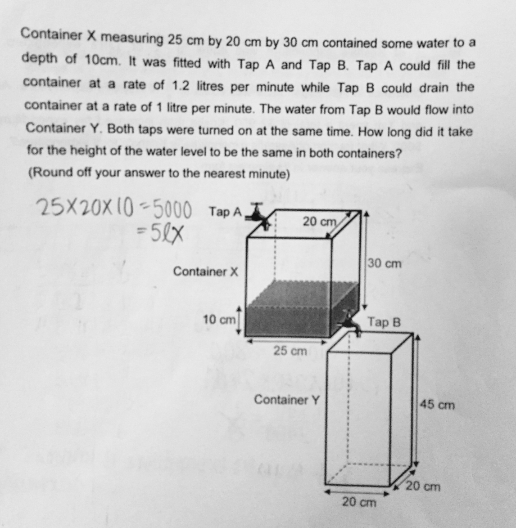# QuestionAnyone help me with attached question

Thanks

height of water contained in Container X ——- 10 cm

In 1 min,

Tap A fills 1.2 litres or 1200 cubic cm and Tap B drains 1 litre or 1000 cubic cm ——–>

Container X ——- + 1200 – 1000 = + 200

Container Y ——- + 1000

200/(25 x 20) = 0.4 (increase in height in Container X in 1 min)
1000/(20 x 20) = 2.5 (increase in height in Container Y in 1 min)
2.5 – 0.4 = 2.1
10/2.1 = 5 (rounded off to the nearest minute)

Ans : 5 min (rounded off to the nearest minute).

I have amended my solution; thank you.

0 Replies 1 Like

Tap A (into X) -> 1200 cm³/min

Tap B (out of X) -> 1000 cm³/min

Nett -> 200 cm³/min

X increased in height -> 200 ÷ base area = 200 ÷ (20 x 25) = 0.4 cm/min

Y increased in height -> 1000 ÷ (20 x 20) = 2.5 cm/min

Time taken for height to be the same -> T min

2.5T = 10 + 0.4T

2.1 T = 10

T = 10 ÷ 2.1 = 4.7619

Time taken = 5 min (round to nearest minute)